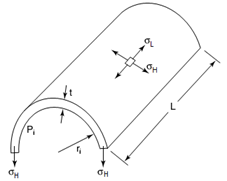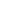### الصفحاتم4 Design مرحلة رابعة

# Thin and Thick Cylinders Experiment مختبر التصميم

Experiment No. 5

Name: Thin and Thick Cylinders

Aim: To study the performance of thin and thick cylinders subjected to internal pressure through the stresses and strain analysis along the length and circumference of cylinders.

Theory:

1-  Thin Cylinder:    When a thin cylinder is subjected to an internal pressure, three mutually perpendicular principal stresses [ hoop stress, longitudinal stress, and radial stress ] are developed in the cylinder material. If the ratio of thickness and the inside diameter of the cylinder is less than (1: 20), membrane theory may be applied and we may assume that the hoop and longitudinal stresses are approximately constant across the wall thickness.

The hoop stress acting on the cylinder wall σH = pi d / 2 t

The longitudinal stress acting on the cylinder wall

σL = pi d / 4t

Where pi is the internal pressure, d is the internal diameter and t  is the wall thickness

Ԑ HO = σ HO / E  ( Open End Cylinder – relation of hoop strain with hoop stress)

Ԑ LO = - ν σ HO / E  ( Open End Cylinder – relation of longitudinal strain with hoop stress)

The principal strain for closed ends cylinder is given by:

Ԑ HC = ( σ H -  ν σ L ) / E

Ԑ LC = ( σ L -  ν σ H ) / E

Strain in direction of σ 1    :    Ԑ 1 =  σ 1 / E  -  ν σ 2  / E

Strain in direction of σ 2    :     Ԑ 2 =  σ 2 / E  -  ν σ 1  / E

Where σ 1 & σ 2 are the principal stresses of the biaxial stresses (σ x = σ L  & σ y = σ H )

σ1, σ2 = ((σx + σy) / 2) ± [(x σy) 2 / 2) + τ 2xy]

After representation of strain on Mohr’s Circle, the other strain can be found as:

Ԑ M = [ (Ԑ 2 + Ԑ 1 ) / 2] + [(Ԑ 1 - Ԑ 2 ) / 2] cos 2θ      (2θ is measured in CCW )

Ԑ N = [ (Ԑ 2 + Ԑ 1 ) / 2] + [(Ԑ 1 - Ԑ 2 ) / 2] cos 2θ      (2θ is measured in CW )

2- Thick Cylinder: The Lamé theory for determination of the stresses in the walls of thick cylindrical shells considers a mutually perpendicular, tri-axial, principal-stress system consisting of the radial, hoop or tangential and longitudinal stresses acting at any element in the wall. For the case of the shell depicted in the attached figure, subjected to internal pressure only, radial stress σ R  , and hoop stress σ H are given by:

Radial Stress = σ R = [ p r i 2 / ( r O2 – r i 2)] * [ 1 – (r O2 / r  2 )]

Hoop Stress  = σ H = [ p r i 2 / ( r O2 – r i 2)] * [ 1 + (r O2 / r  2 )]

The hoop and radial strain will be calculated as: Ԑ H = [σ H - ν σ R ] / E & Ԑ R = [σ R - ν σ H ] / E

Where r is variable between ( r I  &  r O )

Maximum σ R  is at the inner radius = - p  ,  σ R = 0.0 at  the outer radius

Maximum σ H  is the inner radius & minimum  σ R  is at  the outer radius

Maximum shear stress occur at the inner radius = τ = 1.065 p

Also radial & hoop stresses can be calculated from the experimental reading of the strain as:

σ H = [ E / ( 1- ν2 )] * ( Ԑ H  + ν Ԑ R )    &    σ R = [ E / ( 1- ν2 )] * ( Ԑ R  + ν Ԑ H )

In order to get an expression for the longitudinal stress σ L, the shell is considered closed at both ends, as shown in the below figure. Simple consideration of the force equilibrium in the longitudinal direction yields:

σ L = p r i 2 / ( r O2 – r i 2)

Ԑ L = [σ L - ν * (σ H + σ R )] / E

Procedure:

A-   For Thin Cylinder:

1-    Screw the hand wheel to make the thin cylinder as open ends, therefore hoop stress will be generated only.

2-     Switch on the power to thin cylinder and leave it for at least five minutes.

3-     Set all the strain gauge readings to zero.

4-      Pump the hand pump until the pressure is approximately 0.4 MN / m 2. Wait for the six strain gauge reading to stabilize and records these readings.

5-      Carefully increase the pressure step by step up to maximum pressure. At each increment, wait for readings to stabilize and record the six strain gauge readings.

6-    Unscrew the hand wheel to make the thin cylinder as closed ends, so hoop & longitudinal stresses will be generated due to applied internal pressure.

7-    Repeat steps 2, 3, 4, 5, & 8 for the closed ends cylinder. Calculate the principal stresses,  principal strains and draw Mohr’s stress strain circle to calculate the strain of strain gauges 3, 4, & 5.

B-   For Thick Cylinder:

1-    Switch on the power to the thick cylinder and leave at least thirty minutes before starting with the experiment.

2-      Unscrew the pump hand wheel until the pressure gauge shows zero pressure.

3-     Set the strain gauge readings to zero at zero pressure.

4-      Screw in the pump hand wheel until the pressure is approximately 1.0 MPa. Wait a few seconds for readings stabilization and record the strain gauge readings.

5-      Increase the pressure by step of 1.0 MPa up to 7.0 MPa and record the strain gauge readings for each step after stabilization.

Calculations:

A- Thin Cylinder [ D = 86.0 mm, t = 3.0 mm, E = 69 GPa, ν = 0.33  ]

1-    Calculate the hoop stress for the open end cylinder for each pressure.

2-    Draw the relation between the calculate hoop stress and the measured hoop strain ( stain gauge 1 & 6) and get the experimental cylinder modulus of elasticity.

3-    Draw the relation of the measured hoop strain ( strain gauge 1 ) and the longitudinal strain ( strain gauge 2) to get the cylinder material Poisson’s ratio.

4-    Draw the relation of the pressure with the readings of the strain gauges ( 1, 2, 3, 4, 5, & 6). Draw stress & strain Mohr’s Circle for a certain value of pressure.

5-    Calculate the hoop & longitudinal stresses for the closed end cylinder for each pressure. Then calculate the principal stresses & strains.

6-    Draw the relation between the applied pressure and the measured strains ( strain gauges 1, 2, 3, 4, 5, & 6 ) to study the pressure – strain relations.

7-    Draw stress & strain Mohr’s Circle for a certain value of pressure.

B-   Thick cylinder [ d = 37 mm, D = 150 mm, L = 203 mm, E = 73 GPa, ν = 0.33  ]

1-    Draw the relation of the applied pressure with each of the hoop & radial strains that are recorded before for different radius.

2-    Calculate the theoretical hoop and radial stress at all the given radius in the experiment [from the inner radius (18.5 mm ) up to outer radius ( 75 mm )] for internal pressure of 3 & 6 MPa.

3-    Draw the theoretical and derived hoop stresses and the radial stresses with variable radius ( r ), and estimate the difference between theoretical & experimental results.

4-    Calculate the longitudinal stress & strain for all the pressure.

5-    Draw the theoretical and measured longitudinal strain with the variable pressure.

Discussions:

1-    Compare between the theoretical and experimental  of the  thin cylinder results regarding:

1-1     Modulus of elasticity ( E ) and Poisson’s ratio ( ν ).

1-2     Hoop and longitudinal stresses.

1-3     Principal stresses and strain from the Mohr’s Circle and the equations.

2-    Give three applications for the open and closed ends thin cylinders.

3-    Compare between the theoretical and experimental results of the  thick cylinder results regarding:

3-1   The hoop, radial and longitudinal stresses.

3-2   The relation of the hoop & radial strain with the variable radius ( r ).

3-3   The relation of the longitudinal strain with the variable radius ( r ).

4-    Give three applications for the thick cylinders.

5-    Give brief idea about the strain and pressure measurement for the thin and thick cylinders.مهندس ميكانيك
هدفي هو التطوير من ذاتي ثم الاستفادة ثم تطوير غيري